# DIY PCR: Arduino Temperature Sensor

Welcome to the second part of the DIY PCR series! For an overview of what PCR is as well as the goals of this project, check out my introductory post here before reading ahead.

Today’s post will focus on making and installing a thermocouple to keep track of reagent temperature. Ensuring that the temperature is as accurate as possible is important in order for DNA amplification to occur properly. In addition to showing you how to wire all the components together, I’ll also show you the code I used to control everything. Let’s start with the electronics I used.

1. A type k thermocouple: used to accurately measure the temperature
2. AD595 thermocouple amplifier: translates the output of the thermocouple into voltage. The Arduino converts that voltage to a temperature. Without this, any reading we get off the thermocouple will not make sense.
3. Wire
4. Arduino board
5. Breadboard: used to join components together to form a circuit, without having to solder.

On to wiring the components. As someone fairly new to electronics I really had no clue how to go about this. Luckily my googlefu is pretty damn good, so a quick search led me to this datasheet. Below is a diagram that indicates what each of the pins on the amplifier do.

As you can see there are 7 pins on the top and 7 pins on the bottom of the amplifier. Since the pins were nicely labeled it made wiring things up fairly easy.

I started by inserting the amplifier into the breakout board. The pins that are visible in the picture on the left are pins 1–7 and are ordered left to right. I’ll be using the top line of the breadboard as the positive terminal and the bottom line as the negative terminal. To begin with I started with the grounding wires.

As per the wiring diagram, the wires connected to pins 4, 7 and 13 needed to be grounded.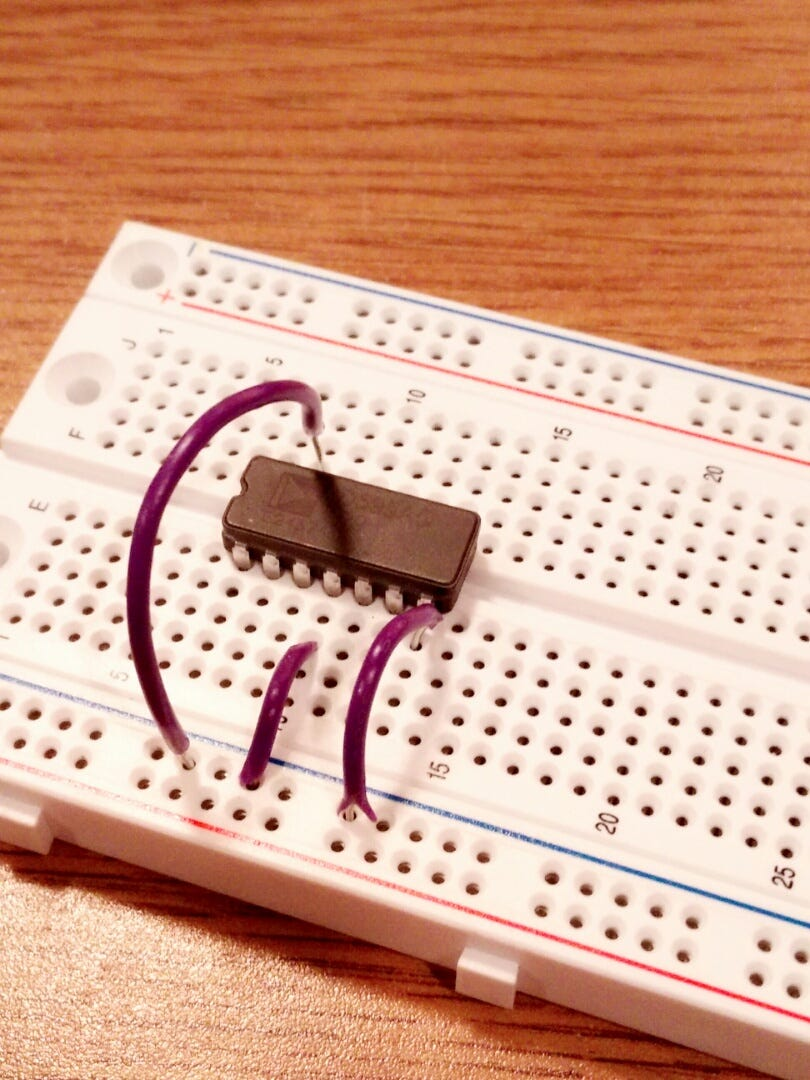Pin’s 4, 7 and 13 grounded

Connecting wires to the positive terminal was up next. In this case just pin 11, which uses 5 volts as power. Lastly, the wire on the left joins pins eight and nine together.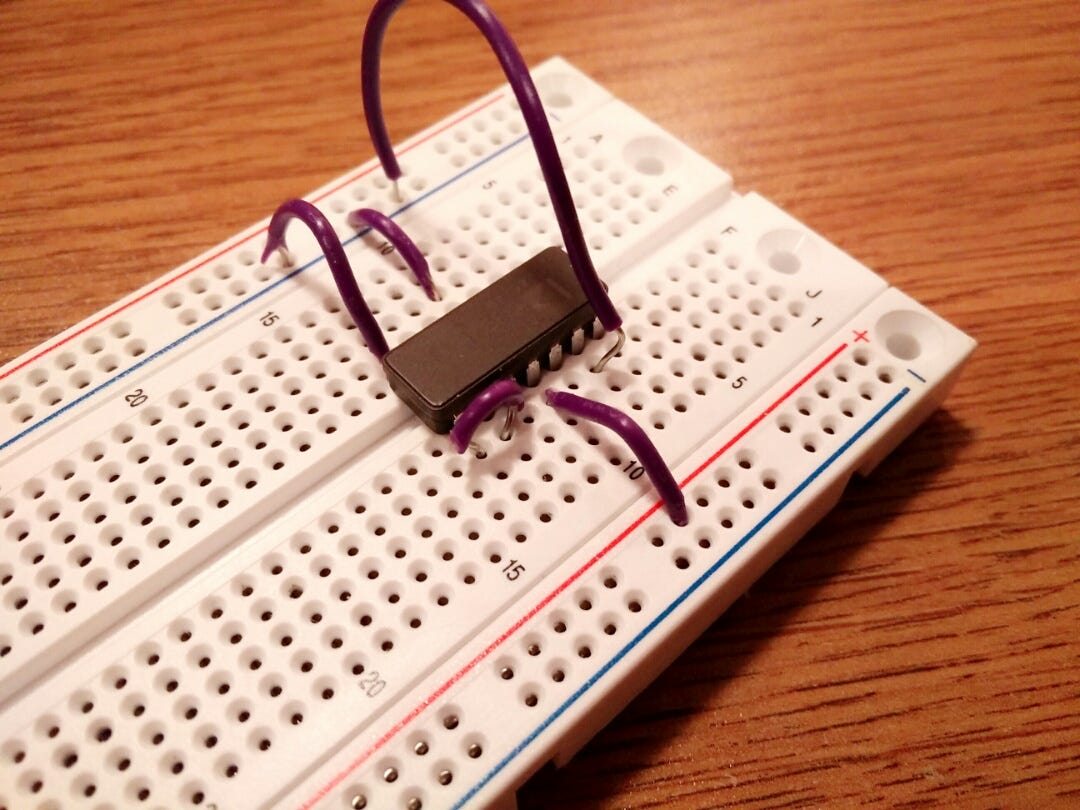Pin 11 connected to positive terminal and pin’s 8 and 9 connected to each other

The last step is to plug in the thermocouple (black and red plugs) into pins 1 and 14. Pin 14 is used for the negative terminal and pin 1 is used for the positive. Finally, a wire connects pin 1 to the negative terminal to ground it.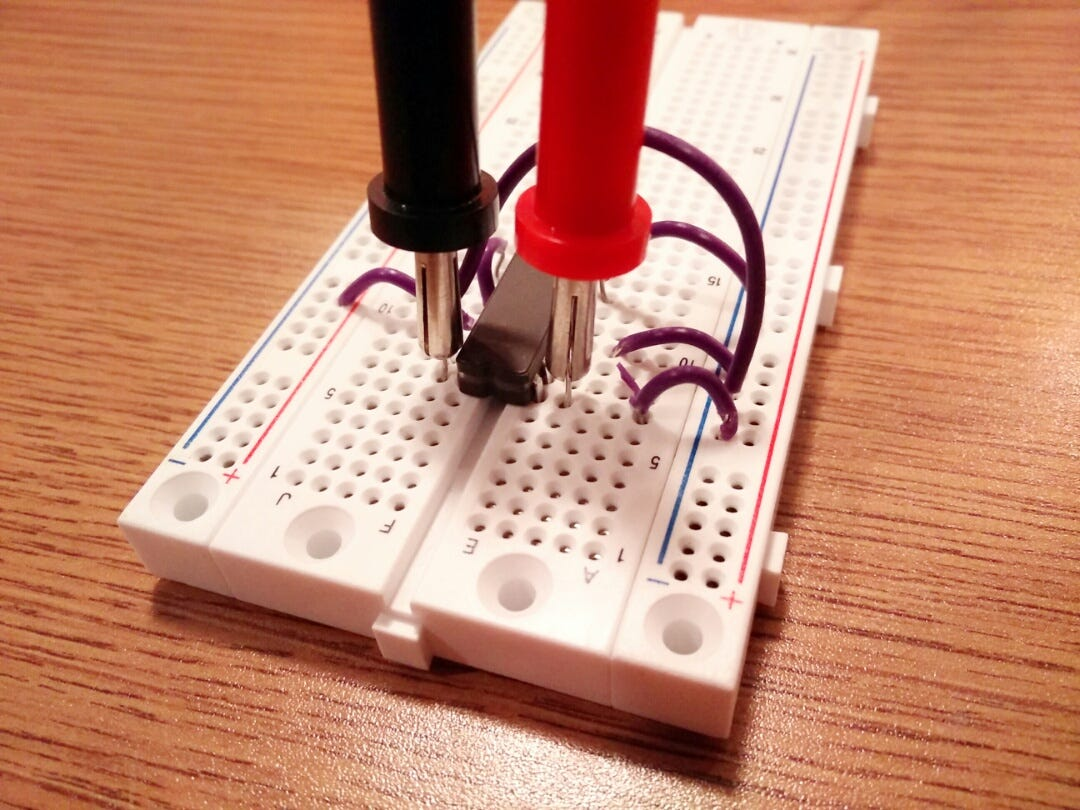Thermocouple (pins 14, 1) and grounding wire (pin 1)

Now that the wires were in the correct position, it was time to attach the breadboard to the Arduino. This would allow the temperature to be displayed on a computer monitor. In the image of the Arduino below, there are 3 wires to make note of. The topmost wire is for power which is plugged into the 5V port. The middle wire is the grounding wire which has been inserted into the GND port. The third and final wire on the bottom is in the A0 port. This allows the Arduino to read in information from the thermocouple/amplifier.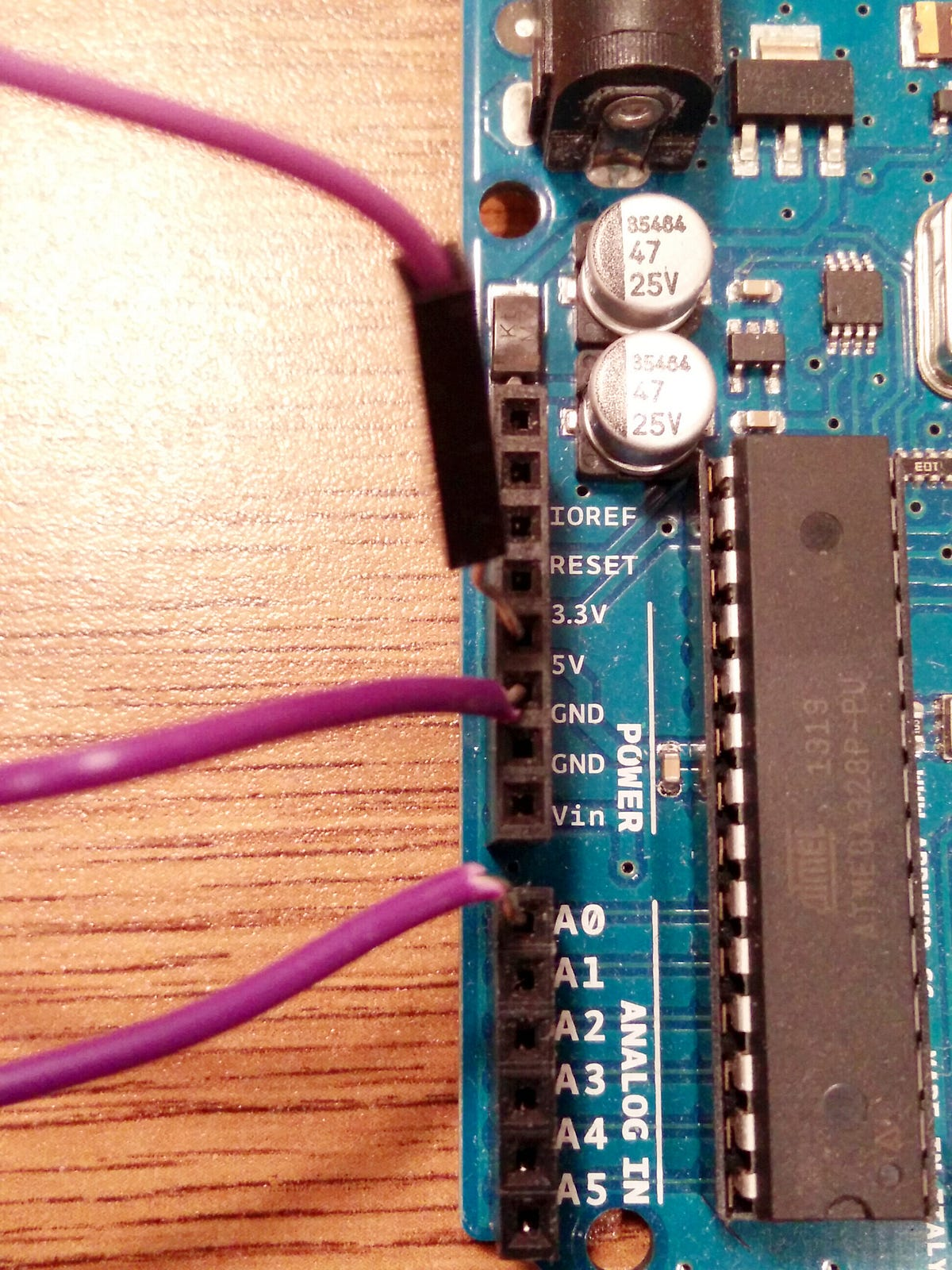Wires connected to 5V, GND and A0 ports

The 5V and GND wires are connected to the positive and negative terminals respectively. The A0 wire will be connected to pin 8 and this wire sends a voltage to the Arduino which will convert it to Celsius.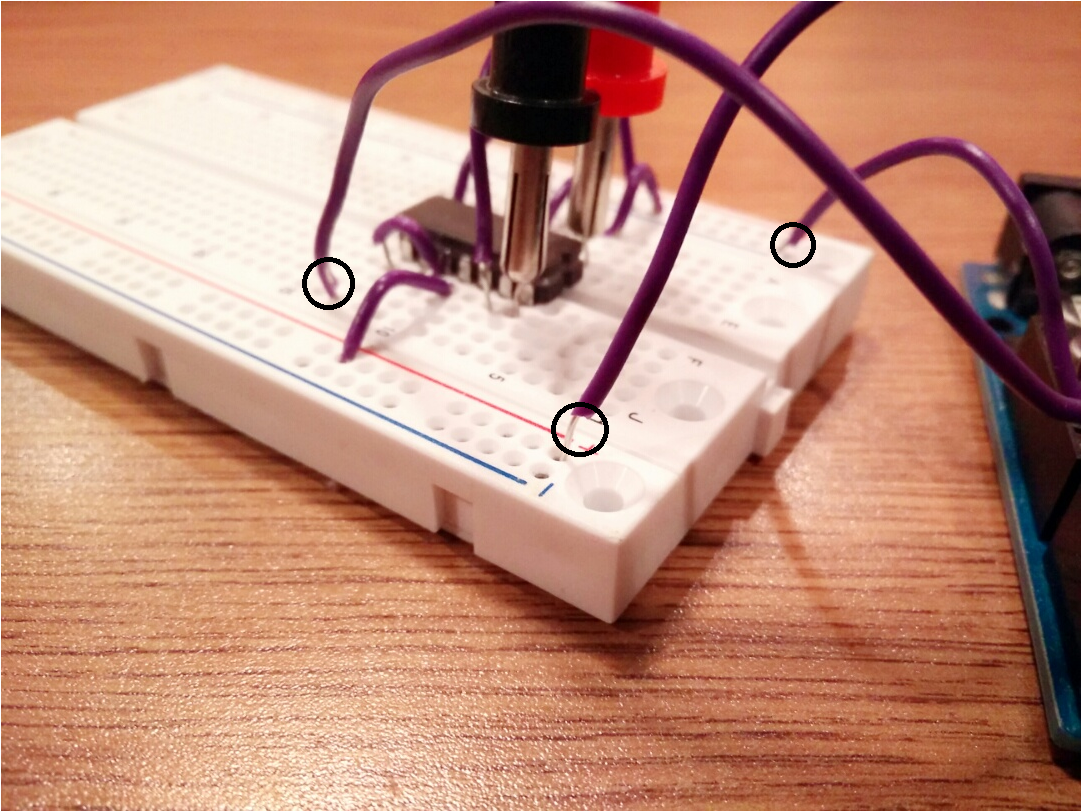(Counter clockwise) A0 wire, power wire, GND wire

Now that the electronic components are set up let’s look at the code needed to control this.

`const int analogInPin = A0;`
`void setup() {     Serial.begin(9600);}`
`void loop() {     sensorValue = analogRead(analogInPin);     int celcius = (((sensorValue / 1023.0) * 5.0) * 100.0);     Serial.print(“Celcius = “ + celcius);    Serial.println();     delay(1000);}`

I started by created a variable analogInPin that stored the location of the input port — in this case A0. The setup function was also used to determine the rate at which the data from the amplifier would be sent to the Arduino.

`const int analogInPin = A0;`
`void setup() {     Serial.begin(9600);}`

The next step was to create a loop function that would constantly get the temperature from the thermocouple. The loop function had to do several things.

1. Read in the voltage value from the thermocouple/amplifier
2. Convert that value to Celsius
3. Pause the loop temporarily

I used analogInPin as a parameter for the analogRead function in order to read in the voltage from the thermocouple. This value was stored in sensorValue.

`sensorValue = analogRead(analogInPin);`

The voltage stored in sensorValue then had to be converted to Celsius. The first step was to divide it by 1023 which is the maximum possible value the amplifier can return. This resulted in the voltage being scaled between 0–1. From there, the now scaled voltage is multiplied by the amount of power being used — in this case 5V and then multiplied again by 100 to scale it to 10mV. From the wiring diagram, pins 8 and 9 on the amplifier were using this scale. The result is this line of code:

`int celcius = (((sensorValue / 1023.0) * 5.0) * 100.0);`

After printing the result to the screen using Serial.print,the delay function was used to pause the program for 1000 milliseconds/1 sec before repeating the code.

`Serial.print(“Celcius = “ + celcius);Serial.println(); delay(1000);`

The end result outputs the current temperature in Celsius, once every second. Now that the wiring and coding as been sorted out, let’s test this and see if it works.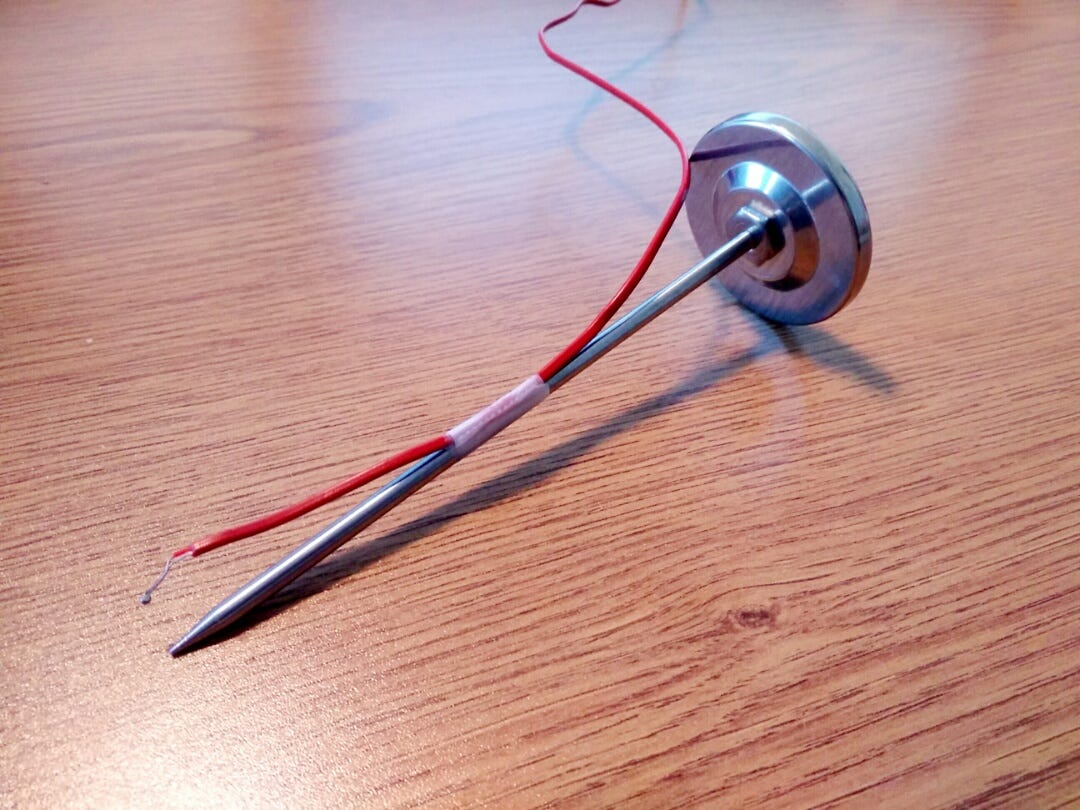(Left Picture) Thermometer (Right Picture) Thermometer and thermocouple taped together

To test the temperature sensor I used two cups- one filled with cool tap water and the other filled with warm tap water. The thermocouple was taped to a thermometer to ensure accurate temperature. For each test, I waited two minutes before comparing the temperatures from the thermometer and thermocouple. The results are recorded below.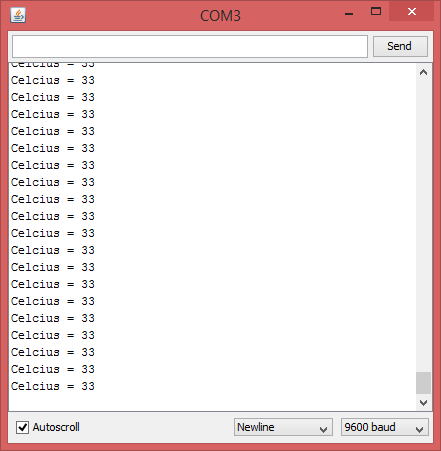(Warm sample) Thermometer temperature at 34 degrees C and thermocouple temperature at 33 degrees C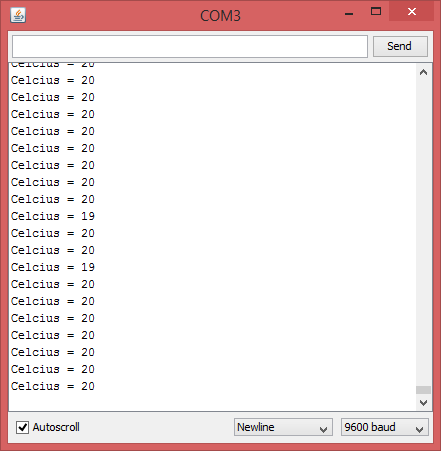(Cool sample) Thermometer temperature at 21 degrees C and thermocouple temperature at 20 degrees C

I should note that the thermocouple has a variance of ±3 degrees Celsius so a discrepancy of 1 degree or so is fine in this case. Since PCR works within a temperature range, i.e. 94–98 degrees Celsius for denaturing DNA, this variance will be taken into consideration in the final code. In short, it looks like the thermocouple is sending back an accurate temperature which we can use!

That just about wraps this post up. In the next one I’ll be going over cooling of the samples and this temperature sensor will definitely come in handy.